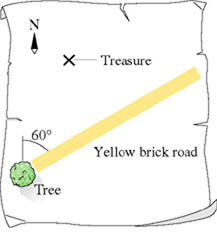# Problem: The treasure map in the figure gives the following directions to the buried treasure: "Start at the old oak tree, walk due north for 510 paces, then due east for 130 paces. Dig." But when you arrive, you find an angry dragon just north of the tree. To avoid the dragon, you set off along the yellow brick road at an angle 60 60 (Page 65) east of north. After walking 360 paces you see an opening through the woods. You may want to review  (Page 65) .For help with math skills, you may want to review:Vector AdditionFor general problem-solving tips and strategies for this topic, you may want to view a Video Tutor Solution of Vectors.a. Which direction should you go to reach the treasure?b. How far should you go to reach the treasure?

###### FREE Expert Solution

To get the vector direction, we use the equation:

$\overline{){\mathbf{tan}}{\mathbit{\theta }}{\mathbf{=}}\frac{{\mathbit{A}}_{\mathbit{y}}}{{\mathbit{A}}_{\mathbit{x}}}}$

Magnitude:

$\overline{)\mathbf{|}\stackrel{\mathbf{⇀}}{\mathbit{A}}\mathbf{|}{\mathbf{=}}\sqrt{{{\mathbit{A}}_{\mathbit{x}}}^{\mathbf{2}}\mathbf{+}{{\mathbit{A}}_{\mathbit{y}}}^{\mathbf{2}}}}$

The vector components are found by:

We let north be positive y-axis and east be positive x-axis.

Now, the co-ordinate of the treasure is:

st = xi + yj

88% (262 ratings)###### Problem Details
The treasure map in the figure gives the following directions to the buried treasure: "Start at the old oak tree, walk due north for 510 paces, then due east for 130 paces. Dig." But when you arrive, you find an angry dragon just north of the tree. To avoid the dragon, you set off along the yellow brick road at an angle 60 60 (Page 65) east of north. After walking 360 paces you see an opening through the woods.You may want to review  (Page 65.

For help with math skills, you may want to review:

For general problem-solving tips and strategies for this topic, you may want to view a Video Tutor Solution of Vectors.

a. Which direction should you go to reach the treasure?

b. How far should you go to reach the treasure?